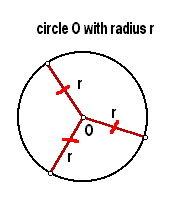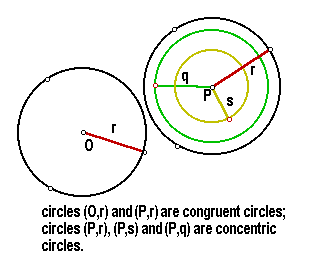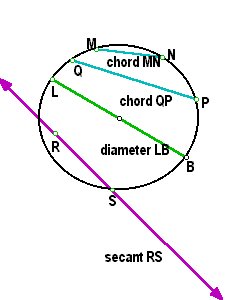BFOIT - Introduction to Computer Programming

# Circles

## Definitions of a Circle, Chords, Tangent and Secant Lines

• This section introduces the properties of the circles. We start with the definition of this geometric object. A circle is the set of all points in a plane at a fixed distance from a fixed point in the plane. The fixed point is called the center of the circle. The fixed distance is called the radius of the circle. A segment from a point of the circle to the center is also called a radius. Usually a circle is named by its center e.g. circle O. Sometimes we are also going to specify the radius r of the circle and name the circle as (O, r).• We say that two circles are congruent if they have the same radius.
• If two or more circles share the same center then they are called concentric circles.• A segment that connects two distinct points on a circle is called a chord.
• A chord that passes through the center of a circle is called a diameter.
• A line that intersects with the circle at exactly two points is called a secant (line).This webpage is from the Penn State University Elementary Geometry Tutorial by Anna Koltsova.This work (BFOIT: Introduction to Computer Programming, by Guy M. Haas),
identified by Berkeley Foundation for Opportunities in IT (BFOIT),
is free of known copyright restrictions.